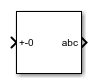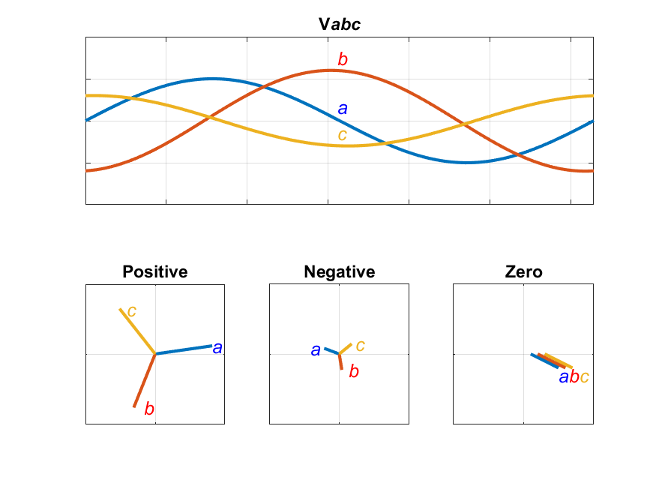# Inverse Symmetrical-Components Transform

Implement +-0 to abc transform

• Library:
• Simscape / Electrical / Control / Mathematical Transforms

•## Description

The Inverse Symmetrical-Components Transform block implements an inverse symmetrical transform of a positive, negative, and zero phasor. The transform splits a symmetrical set of three phasors into the equivalent unbalanced set of a, b, and c phasors.

Use this transform to regenerate a three-phase signal from a system that was decoupled using the Symmetrical-Components Transform block.

Use the `Power invariant` property to choose between the Fortescue transform, and the alternative, power-invariant version.

### Equations

The inverse symmetrical-components transform regenerates an unbalanced three-phase signal [Va,Vb,Vc] from the a components of a balanced set of phasors [Va+,Va-,Va0], given in the +-0 domain:

`$\left[\begin{array}{c}{V}_{a}\\ {V}_{b}\\ {V}_{c}\end{array}\right]=\frac{1}{K}\left[\begin{array}{ccc}1& 1& 1\\ {a}^{2}& a& 1\\ a& {a}^{2}& 1\end{array}\right]\left[\begin{array}{c}{V}_{a+}\\ {V}_{a-}\\ {V}_{a0}\end{array}\right].$`

where, a is the complex rotation operator

`$a={e}^{2\pi i/3},$`

and K is the constant that determines the type of transform:

If the transform was performed using the power-invariant option, enable the `Power invariant` property to select the power-invariant inverse transform and regenerate the correct abc signal.

### Symmetrical-Components Transform

The symmetrical-components transform separates an unbalanced three-phase signal given in phasor quantities into three balanced sets of phasors:

`$\left[\begin{array}{c}{v}_{a}\\ {v}_{b}\\ {v}_{c}\end{array}\right]=\left[\begin{array}{c}{v}_{a+}\\ {v}_{b+}\\ {v}_{c+}\end{array}\right]+\left[\begin{array}{c}{v}_{a-}\\ {v}_{b-}\\ {v}_{c-}\end{array}\right]+\left[\begin{array}{c}{v}_{a0}\\ {v}_{b0}\\ {v}_{c0}\end{array}\right],$`

where:

• va, vb, and vc make up the original, unbalanced set of phasors.

• va+, vb+, and vc+ make up the balanced, positive set of phasors.

• va-, vb-, and vc- make up the balanced, negative set of phasors.

• va0, vb0, and vc0 make up the balanced, zero set of phasors.

The symmetrical-components transform calculates the symmetric a-phase as:

`$\left[\begin{array}{c}{V}_{a+}\\ {V}_{a-}\\ {V}_{a0}\end{array}\right]=\frac{K}{3}\left[\begin{array}{ccc}1& a& {a}^{2}\\ 1& {a}^{2}& a\\ 1& 1& 1\end{array}\right]\left[\begin{array}{c}{V}_{a}\\ {V}_{b}\\ {V}_{c}\end{array}\right].$`

Because the remaining two sets of symmetrical phasors are not often used in calculation, the transformation only generates the first set. However, you can calculate the b- and c-sets in terms of simple rotations of the first:

`$\left[\begin{array}{c}{V}_{b+}\\ {V}_{b-}\\ {V}_{b0}\end{array}\right]=\left[\begin{array}{ccc}{a}^{2}& 0& 0\\ 0& a& 0\\ 0& 0& 1\end{array}\right]\left[\begin{array}{c}{V}_{a+}\\ {V}_{a-}\\ {V}_{a0}\end{array}\right],$`

and

`$\left[\begin{array}{c}{V}_{c+}\\ {V}_{c-}\\ {V}_{c0}\end{array}\right]=\left[\begin{array}{ccc}a& 0& 0\\ 0& {a}^{2}& 0\\ 0& 0& 1\end{array}\right]\left[\begin{array}{c}{V}_{a+}\\ {V}_{a-}\\ {V}_{a0}\end{array}\right].$`

### Operating Principle

The three sets of balanced phasors generated by the symmetrical-components transform have the following properties:

• The positive set has the same order as the unbalanced set of phasors a-b-c.

• The negative set has the opposite order as the unbalanced set of phasors a-c-b.

• The zero set has no order because all three phasor angles are equal.

This diagram visualizes the separation performed by the transform.In the diagram, the top axis shows an unbalanced three-phase signal with components a, b, and c. The bottom set of axes separates the three-phase signal into symmetrical positive, negative, and zero phasors.

Observe that in each case, the a, b, and c components are symmetrical and are separated by:

• +120 degrees for the positive set.

• -120 degrees for the negative set.

• 0 degrees for the zero set.

## Ports

### Input

expand all

Positive, negative, and zero a phasors given as a complex signal. Use the rotations given in the Symmetrical-Components Transform section to compute the b and c phasor sets.

Data Types: `single` | `double`

### Output

expand all

Regenerated three-phase set of unbalanced phasors, output as a complex signal.

Data Types: `single` | `double`

## Parameters

expand all

Power invariant toggle. Select this parameter to use the power-invariant alternative of the original Fortescue transform.

 Anderson, P. M. Analysis of Faulted Power Systems. Hoboken, NJ: Wiley-IEEE Press, 1995.